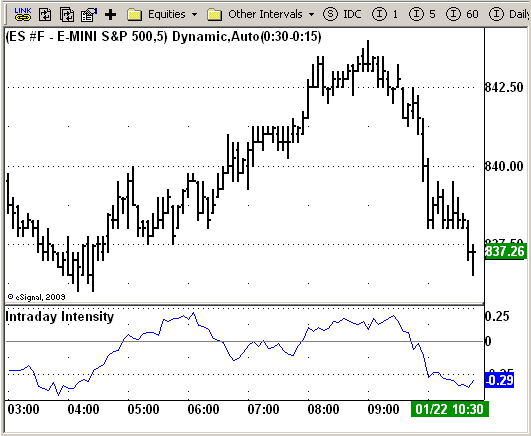ICE Data Services -

EFSLibrary - Discussion Board

Description:

mula Parameters:

• Length : 20

Notes:
This indicator is created by John Bollinger. It is calculated according to this formula:

`IntradayIntensity = SUM{((2 * Close-High-Low)/(High-Low)) * Volume} / SUM{Volume}`EFS Code:

```/*********************************
Provided By:
eSignal (Copyright c eSignal), a division of Interactive Data
Formula Script (EFS) is for educational purposes only and may be
modified and saved under a new file name.  eSignal is not responsible
for the functionality once modified.  eSignal reserves the right
to modify and overwrite this EFS file with each new release.

Description:

Version:            1.0  01/19/2009

Formula Parameters:                     Default:
Length                              20

Notes:
This indicator is created by John Bollinger. It is calculated according to this formula:
IntradayIntensity = SUM{((2 * Close-High-Low)/(High-Low)) * Volume} / SUM{Volume}

**********************************/

var fpArray = new Array();
var bInit = false;

function preMain() {
setDefaultBarFgColor(Color.blue, 0);

var x=0;
fpArray[x] = new FunctionParameter("Length", FunctionParameter.NUMBER);
with(fpArray[x++]){
setLowerLimit(1);
setDefault(20);
}
}

var xIntensity = null;

function main(Length) {
var nState = getBarState();
if (nState == BARSTATE_ALLBARS) {
if (Length == null) Length = 20;
}
if ( bInit == false ) {
xIntensity = efsInternal("Calc_Value", Length);
bInit = true;
}
if (getCurrentBarCount() < Length) return;
return xIntensity.getValue(0);
}

function Calc_Value(nLength) {
var nRes = 0;
var i = 0;
var	xSum = 0;
var	ySum = 0;
for(i = -nLength; i <= 0; i++){
if(high(i) - low(i) != 0)
xSum += (2 * close(i) - high(i) - low(i)) *  volume(i) / (high(i) - low(i));
ySum += volume(i);
}
nRes = xSum / ySum;
if (nRes == null) nRes = 1;
return nRes;
}```